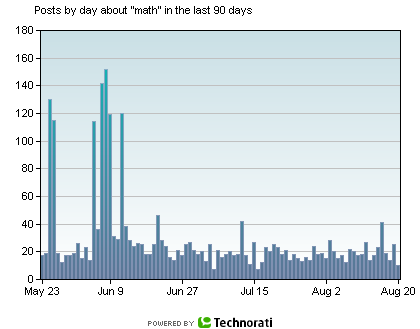Search IntMath
Close

# Why the surge of interest in math?

By Murray Bourne, 21 Aug 2006

I did a Technorati search on the term "math". I am intrigued why there was a surge of interest in posts containing "math" on May 25/26 and during the first 10 days of June 2006.

Was it the beginning of a new series of Numb3rs, or something?

Here is the graph showing the surge: (Source: Technorati, 21 Aug 2006)Unfortunately, Technorati no longer provides these charts. It's a shame.

Alternatively, here's the result for "math" on Google Trends.

Be the first to comment below.

### Comment Preview

HTML: You can use simple tags like <b>, <a href="...">, etc.

To enter math, you can can either:

1. Use simple calculator-like input in the following format (surround your math in backticks, or qq on tablet or phone):
a^2 = sqrt(b^2 + c^2)
(See more on ASCIIMath syntax); or
2. Use simple LaTeX in the following format. Surround your math with $$ and $$.
$$\int g dx = \sqrt{\frac{a}{b}}$$
(This is standard simple LaTeX.)

NOTE: You can mix both types of math entry in your comment.